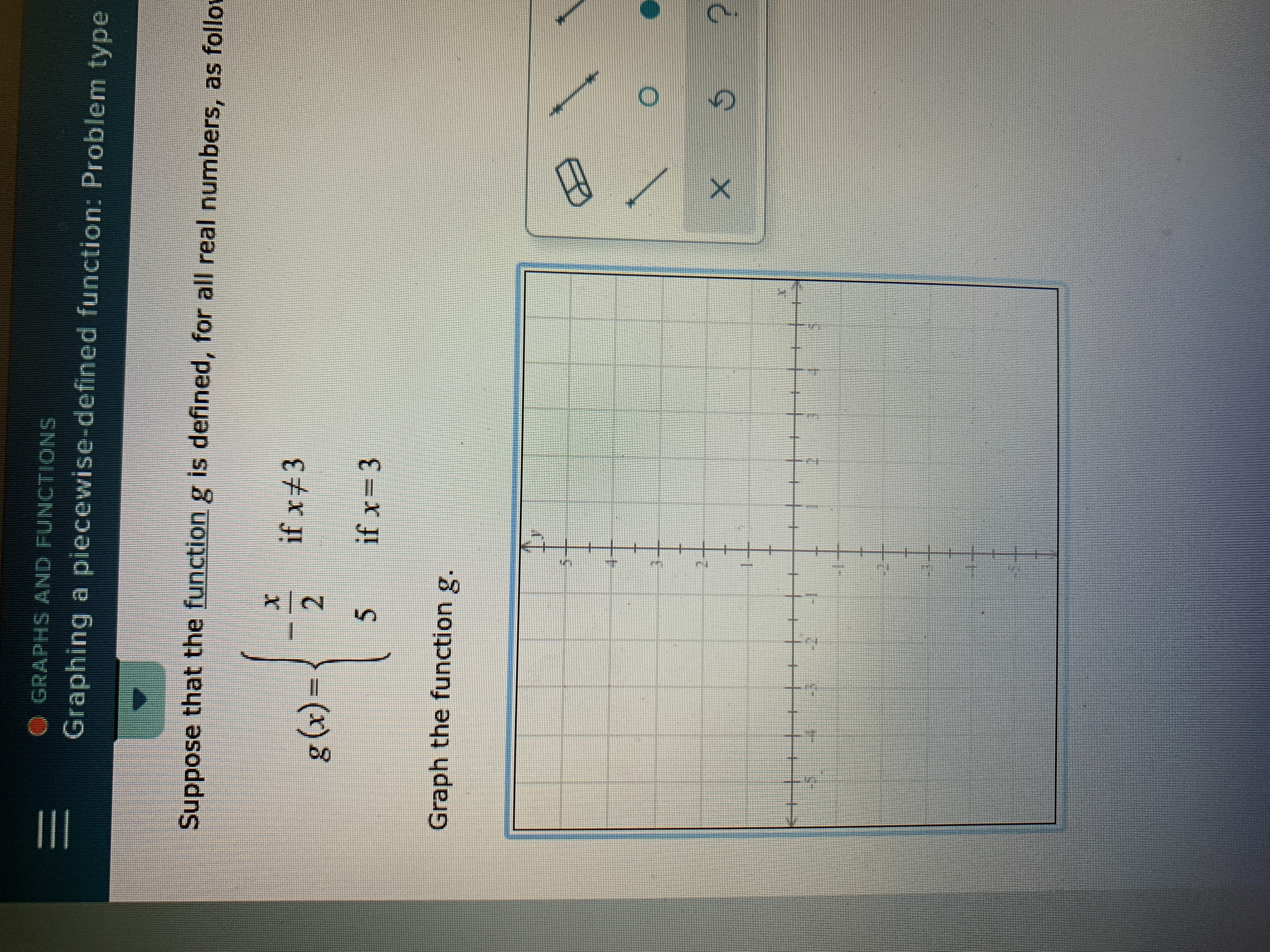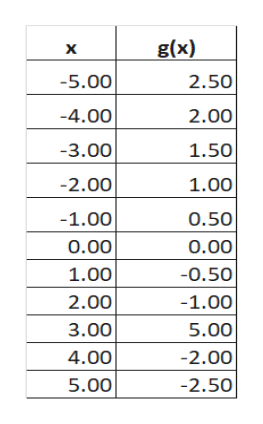# GRAPHS AND FUNCTIONSGraphing a piecewise-defined function: Problem typeSuppose that the function g is defined, for all real numbers, as followif x32g (x)if x=35Graph the function g.

Questionhelp_outlineImage TranscriptioncloseGRAPHS AND FUNCTIONS Graphing a piecewise-defined function: Problem type Suppose that the function g is defined, for all real numbers, as follow if x3 2 g (x) if x=3 5 Graph the function g. fullscreen
check_circleExpert Solution
Step 1

The function has a break at x = 3. We need to evaluate if the function is continuous at x = 3.

Step 2

As we approach x = 3 from the left hand side we get, g(3 - h) = - (3 - h) / 2 = - 3 / 2

As we approach x = 3 from the right hand side we get, g(3 + h) = - (3+ h) / 2 = - 3 / 2

g(3) = 5

Since the left hand limit = right hand limit, but they ae not equal to the value of the function at the given point, hence the given function is not continuous at x = 3.

Step 3

The table for plot will be as show on...help_outlineImage Transcriptioncloseg(x) X 2.50 -5.00 -4.00 2.00 -3.00 1.50 -2.00 1.00 -1.00 0.50 O.00 0.00 1.00 -0.50 2.00 -1.00 3.00 5.00 4.00 -2.00 5.00 -2.50 fullscreen

### Want to see the full answer?

See Solution

#### Want to see this answer and more?

Solutions are written by subject experts who are available 24/7. Questions are typically answered within 1 hour*

See Solution
*Response times may vary by subject and question
Tagged in

### Functions